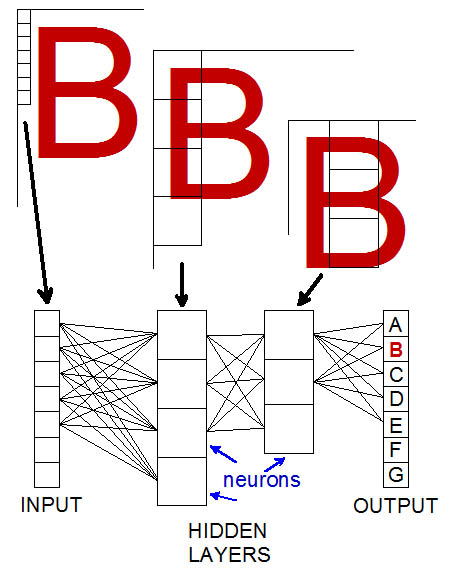# Definition: convolutional neural network

A widely used neural network that recognizes patterns. The convolutional neural network (CNN) breaks the input image into pixels and connects them to a series of neuron layers, each of which sees the image in a different location. The output layer is the best interpretation of the input. See neural network, machine learning and AI.Character Recognition
This simplistic example shows how the letter "B" is recognized. Each layer examines another part of the image, and every input pixel (greatly exaggerated here) is connected to every neuron in the next layer and so on all the way to the output. The interconnections are mathematically weighted and computed in each layer.There Are Many Kinds of CNNs
Neural networks can be complex. These are examples of convolutional architectures from the Asimov Institute. (Images courtesy of Fjodor van Veen and Stefan Leijnen (2019). The Neural Network Zoo, www.asimovinstitute.org/neural-network-zoo)